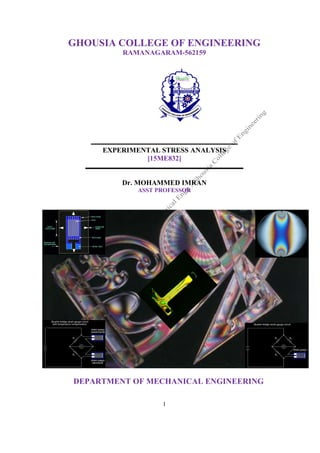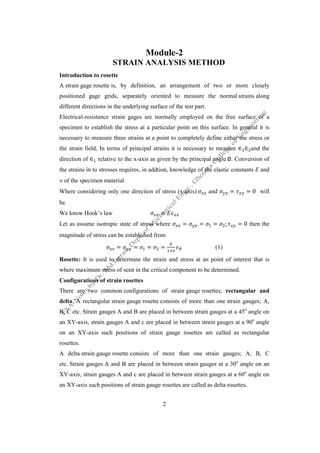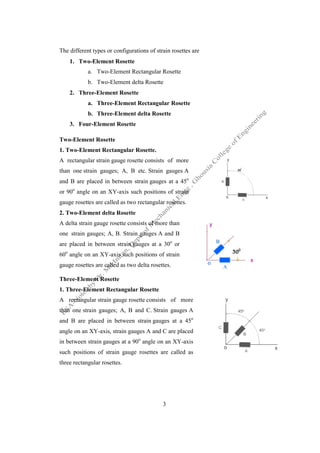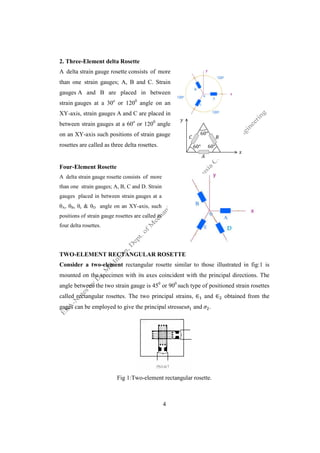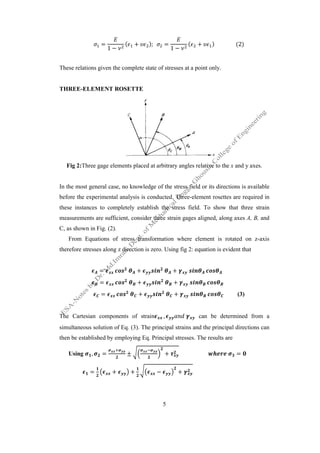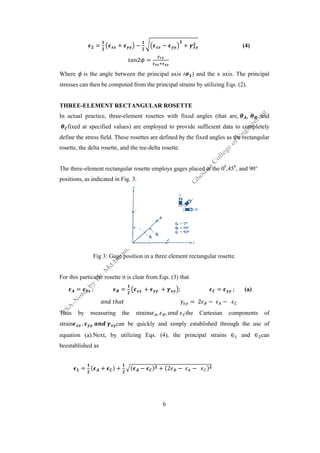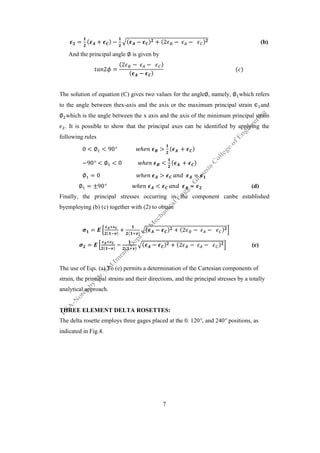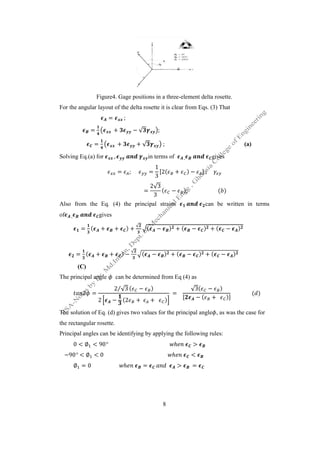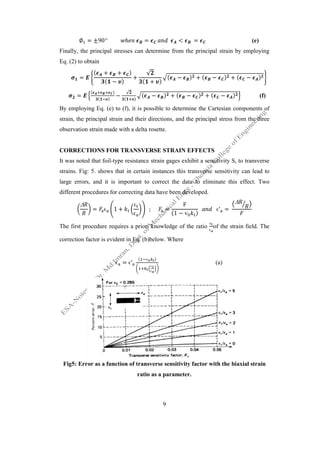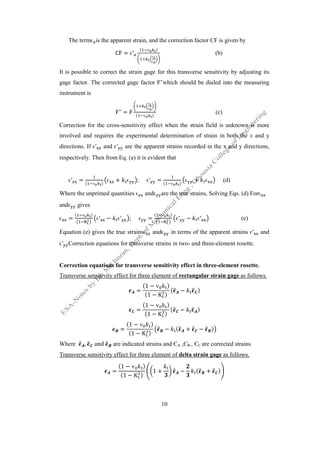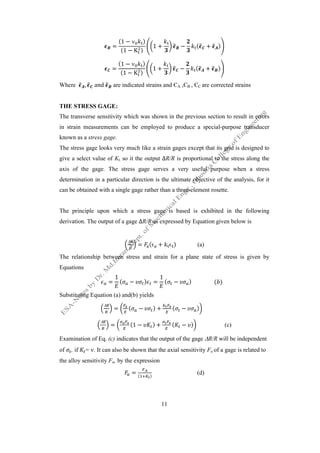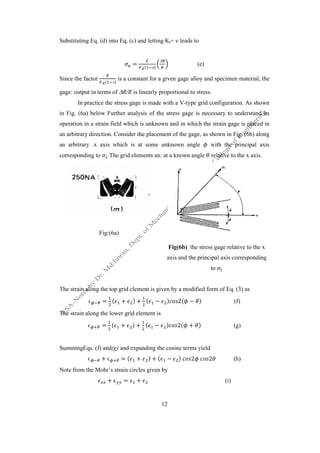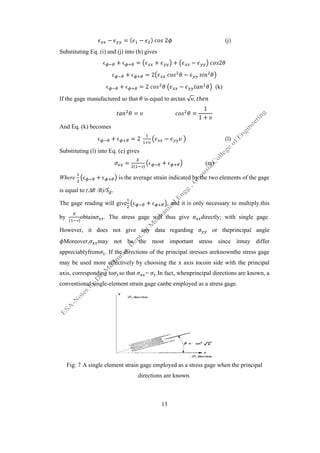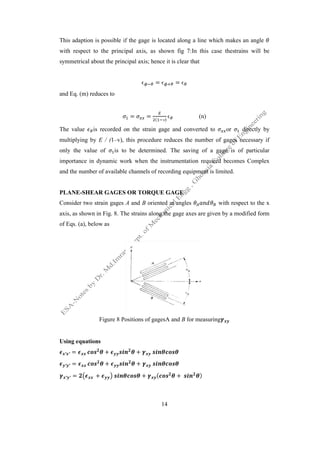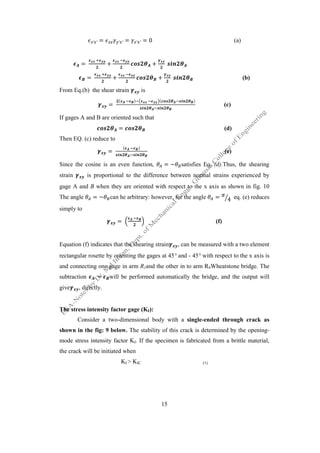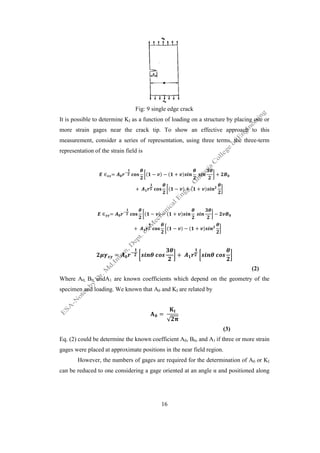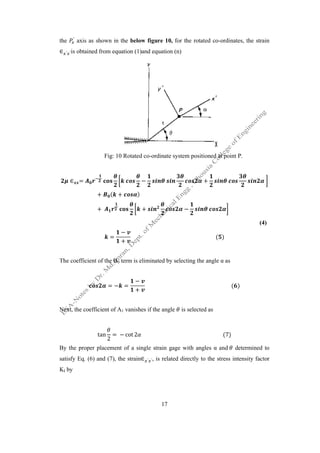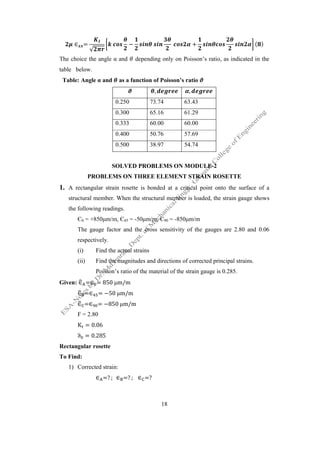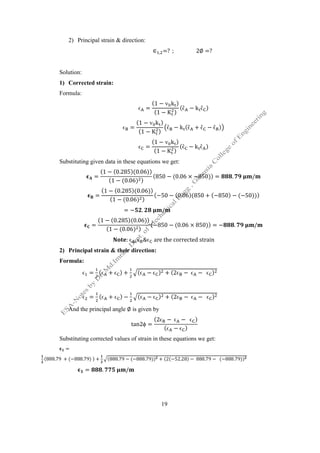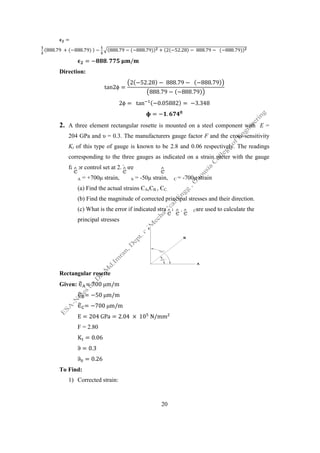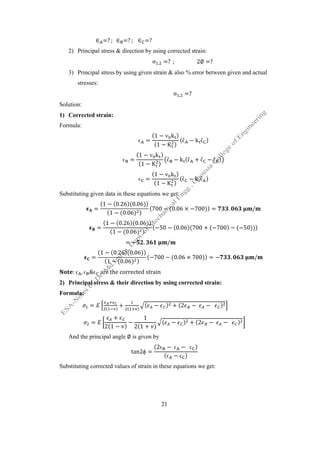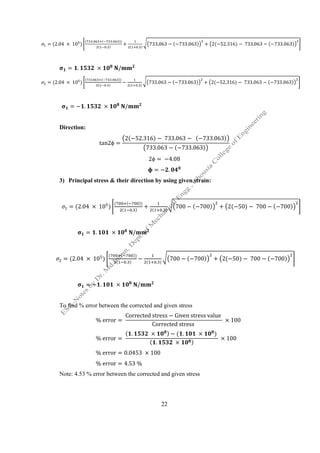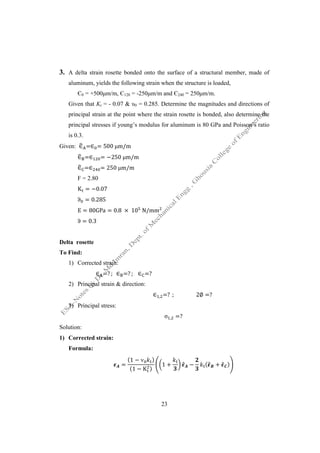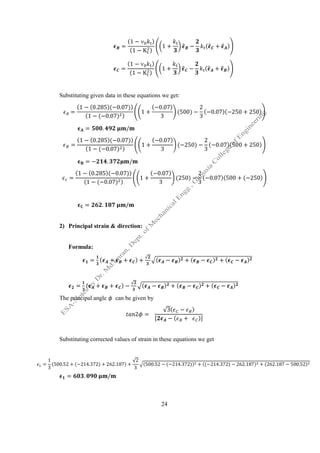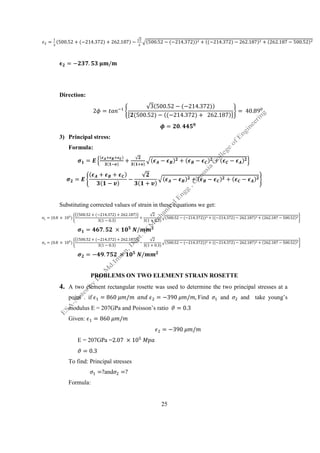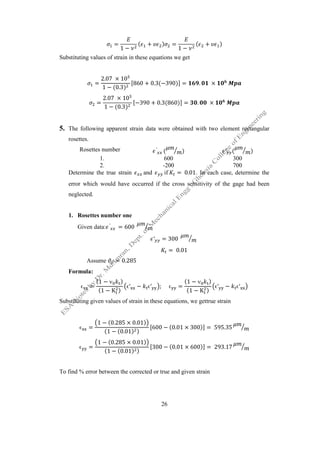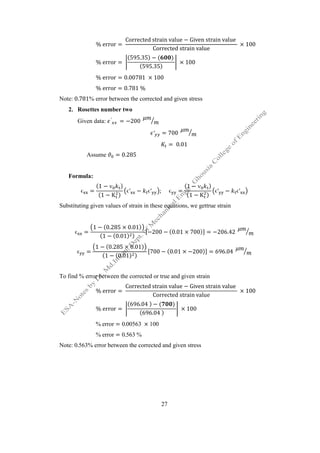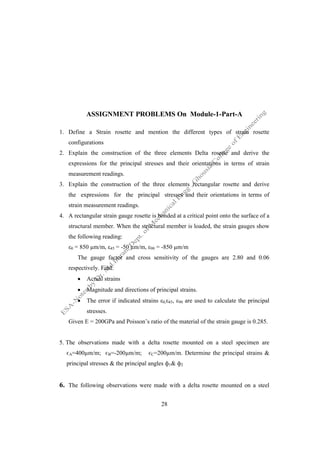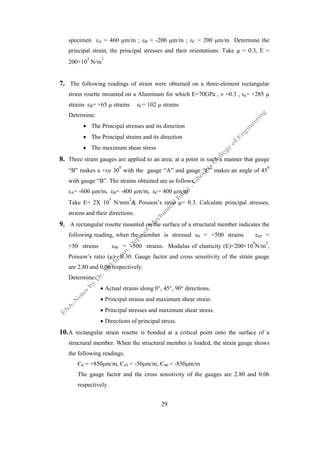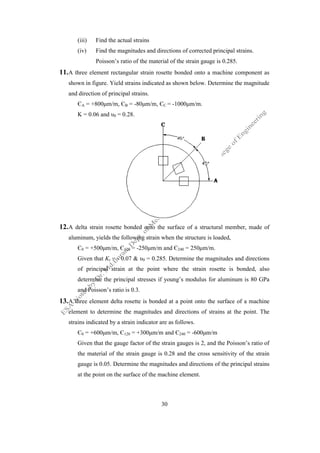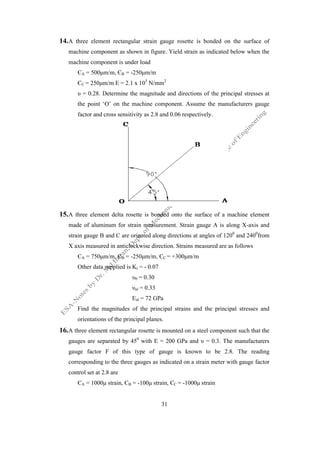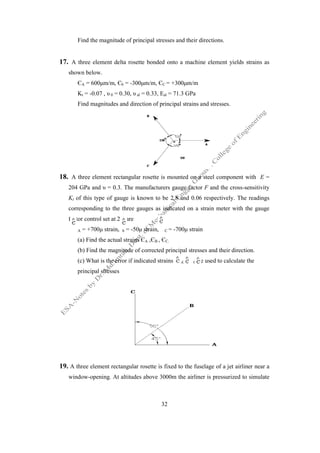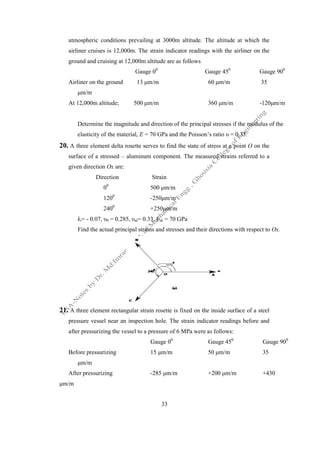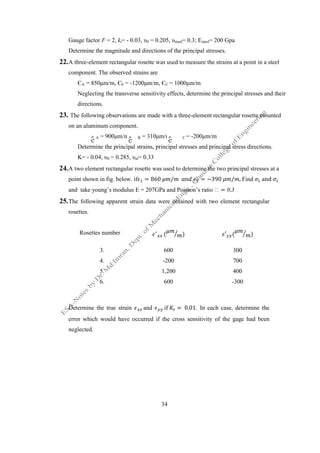Próximo SlideShare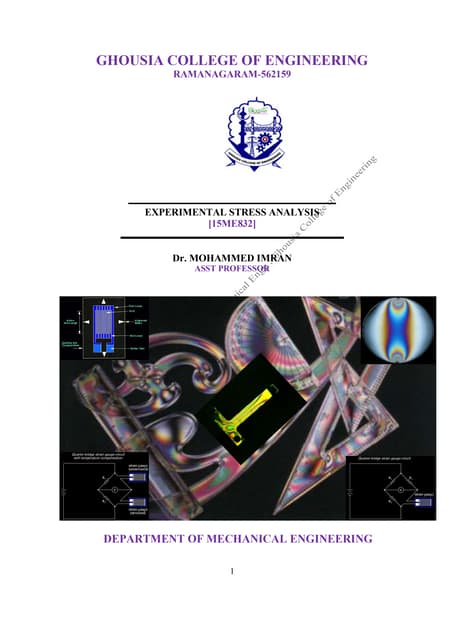ESA Module 1 Part-B ME832. by Dr. Mohammed Imran
Cargando en ... 3
1 de 34

### ESA Module 2 ME832. by Dr. Mohammed Imran

1. 1 GHOUSIA COLLEGE OF ENGINEERING RAMANAGARAM-562159 EXPERIMENTAL STRESS ANALYSIS [15ME832] Dr. MOHAMMED IMRAN ASST PROFESSOR DEPARTMENT OF MECHANICAL ENGINEERING
2. 2 Module-2 STRAIN ANALYSIS METHOD Introduction to rosette A strain gage rosette is, by definition, an arrangement of two or more closely positioned gage grids, separately oriented to measure the normal strains along different directions in the underlying surface of the test part. Electrical-resistance strain gages are normally employed on the free surface of a specimen to establish the stress at a particular point on this surface. In general it is necessary to measure three strains at a point to completely define either the stress or the strain field, In terms of principal strains it is necessary to measure ∈ ∈ and the direction of ∈ relative to the x-axis as given by the principal angle ∅. Conversion of the strains in to stresses requires, in addtion, knowledge of the elastic constants E and  of the specimen material. Where considering only one direction of stress (x-axis) and = = 0 will be We know Hook’s law = Let as assume isotropic state of stress where = = = ; = 0 then the magnitude of stress can be established from = = = = (1) Rosette: It is used to determine the strain and stress at an point of interest that is where maximum stress of seen in the critical component to be determined. Configurations of strain rosettes There are two common configurations of strain gauge rosettes; rectangular and delta. A rectangular strain gauge rosette consists of more than one strain gauges; A, B, C etc. Strain gauges A and B are placed in between strain gauges at a 45o angle on an XY-axis, strain gauges A and c are placed in between strain gauges at a 90o angle on an XY-axis such positions of strain gauge rosettes are called as rectangular rosettes. A delta strain gauge rosette consists of more than one strain gauges; A, B, C etc. Strain gauges A and B are placed in between strain gauges at a 30o angle on an XY-axis, strain gauges A and c are placed in between strain gauges at a 60o angle on an XY-axis such positions of strain gauge rosettes are called as delta rosettes.
3. 3 The different types or configurations of strain rosettes are 1. Two-Element Rosette a. Two-Element Rectangular Rosette b. Two-Element delta Rosette 2. Three-Element Rosette a. Three-Element Rectangular Rosette b. Three-Element delta Rosette 3. Four-Element Rosette Two-Element Rosette 1. Two-Element Rectangular Rosette. A rectangular strain gauge rosette consists of more than one strain gauges; A, B etc. Strain gauges A and B are placed in between strain gauges at a 45o or 90o angle on an XY-axis such positions of strain gauge rosettes are called as two rectangular rosettes. 2. Two-Element delta Rosette A delta strain gauge rosette consists of more than one strain gauges; A, B. Strain gauges A and B are placed in between strain gauges at a 30o or 60o angle on an XY-axis such positions of strain gauge rosettes are called as two delta rosettes. Three-Element Rosette 1. Three-Element Rectangular Rosette A rectangular strain gauge rosette consists of more than one strain gauges; A, B and C. Strain gauges A and B are placed in between strain gauges at a 45o angle on an XY-axis, strain gauges A and C are placed in between strain gauges at a 90o angle on an XY-axis such positions of strain gauge rosettes are called as three rectangular rosettes.
4. 4 2. Three-Element delta Rosette A delta strain gauge rosette consists of more than one strain gauges; A, B and C. Strain gauges A and B are placed in between strain gauges at a 30o or 1200 angle on an XY-axis, strain gauges A and C are placed in between strain gauges at a 60o or 1200 angle on an XY-axis such positions of strain gauge rosettes are called as three delta rosettes. Four-Element Rosette A delta strain gauge rosette consists of more than one strain gauges; A, B, C and D. Strain gauges placed in between strain gauges at a A, B, c & D angle on an XY-axis, such positions of strain gauge rosettes are called as four delta rosettes. TWO-ELEMENT RECTANGULAR ROSETTE Consider a two-element rectangular rosette similar to those illustrated in fig:1 is mounted on the specimen with its axes coincident with the principal directions. The angle between the two strain gauge is 450 or 900 such type of positioned strain rosettes called rectangular rosettes. The two principal strains, ∈ and ∈ obtained from the gages can be employed to give the principal stresses and . Fig 1:Two-element rectangular rosette.
5. 5 = 1 − ( + ); = 1 − ( + ) (2) These relations given the complete state of stresses at a point only. THREE-ELEMENT ROSETTE Fig 2:Three gage elements placed at arbitrary angles relative to the x and y axes. In the most general case, no knowledge of the stress field or its directions is available before the experimental analysis is conducted. Three-element rosettes are required in these instances to completely establish the stress field. To show that three strain measurements are sufficient, consider three strain gages aligned, along axes A, B, and C, as shown in Fig. (2). From Equations of stress transformation where element is rotated on z-axis therefore stresses along z direction is zero. Using fig 2: equation is evident that = + + = + + = + + (3) The Cartesian components of strain , can be determined from a simultaneous solution of Eq. (3). The principal strains and the principal directions can then be established by employing Eq. Principal stresses. The results are Using , = ± + = = + + − +
6. = + Where is the angle between the principal axis stresses can then be computed from the principal strains by utilizing Eqs. (2). THREE-ELEMENT RECTANGULAR ROSETTE In actual practice, three- fixed at specified values) are employed to provide sufficient data to completely define the stress field. These rosettes are defined by the fixed angles as the rectangular rosette, the delta rosette, and the tee The three-element rectangular rosette employs gages placed at the 0 positions, as indicated in Fig. 3. Fig 3: Gage position in a three element rectangular rosette. For this particular rosette it is clear from Eqs. (3) that = ; = ℎ Thus by measuring the strains strain , can be quickly and simply established through the use of equation (a).Next, by utilizing Eqs. (4), the principal strains beestablished as = ( + ) + 6 − − + 2 = is the angle between the principal axis ( ) and the x axis. The principal stresses can then be computed from the principal strains by utilizing Eqs. (2). ELEMENT RECTANGULAR ROSETTE -element rosettes with fixed angles (that are, fixed at specified values) are employed to provide sufficient data to completely define the stress field. These rosettes are defined by the fixed angles as the rectangular rosette, the delta rosette, and the tee-delta rosette. element rectangular rosette employs gages placed at the 00 ,45 positions, as indicated in Fig. 3. Fig 3: Gage position in a three element rectangular rosette. For this particular rosette it is clear from Eqs. (3) that = + + ; = = 2 − − Thus by measuring the strains , , the Cartesian components of can be quickly and simply established through the use of equation (a).Next, by utilizing Eqs. (4), the principal strains ∈ ) ( − ) + (2 − − ) (4) ) and the x axis. The principal stresses can then be computed from the principal strains by utilizing Eqs. (2). ngles (that are, , and fixed at specified values) are employed to provide sufficient data to completely define the stress field. These rosettes are defined by the fixed angles as the rectangular ,450 , and 90° Fig 3: Gage position in a three element rectangular rosette. ; (a) components of can be quickly and simply established through the use of ∈ and ∈ can
7. 7 = ( + ) − ( − ) + (2 − − ) (b) And the principal angle ∅ is given by 2 = (2 − − ) ( − ) ( ) The solution of equation (C) gives two values for the angle∅, namely, ∅ which refers to the angle between thex-axis and the axis or the maximum principal strain ∈ and ∅ which is the angle between the x axis and the axis of the minimum principal strain . It is possible to show that the principal axes can be identified by applying the following rules 0 < ∅ < 90° ℎ > ( + ) −90° < ∅ < 0 ℎ < ( + ) ∅ = 0 ℎ > = ∅ = ±90° ℎ < = (d) Finally, the principal stresses occurring in the component canbe established byemploying (b) (c) together with (2) to obtain = ( ) + ( ) ( − ) + (2 − − ) = ( ) − ( ) ( − ) + (2 − − ) (e) The use of Eqs. (a) To (e) permits a determination of the Cartesian components of strain, the principal strains and their directions, and the principal stresses by a totally analytical approach. THREE ELEMENT DELTA ROSETTES: The delta rosette employs three gages placed at the 0. 120°, and 240° positions, as indicated in Fig.4.
8. Figure4. Gage positions in a three For the angular layout of the delta rosette it is clear from Eqs. (3) That = = + = + Solving Eq.(a) for , = Also from the Eq. (4) the principal strains of , gives = ( + + = ( + + ) (C) The principal angle can be determined from Eq (4) as 2 = 2 √⁄ 2 − The solution of Eq. (d) gives two values for the principal angle the rectangular rosette. Principal angles can be identifying by applying the following rules: 0 < ∅ < 90° −90° < ∅ < 0 ∅ = 0 8 Figure4. Gage positions in a three-element delta rosette. For the angular layout of the delta rosette it is clear from Eqs. (3) That ; − √ ; + √ ; in terms of , gives ; = 1 3 [2( + ) − ] ; = 2√3 3 ( − ); ( ) Also from the Eq. (4) the principal strains can be written in terms ) + √ ( − ) + ( − ) + ( − ) − √ ( − ) + ( − ) + ( − can be determined from Eq (4) as √3 ( − ) (2 + + ) = √3( − ) [ − ( + )] The solution of Eq. (d) gives two values for the principal angle , as was the case for Principal angles can be identifying by applying the following rules: ℎ > ℎ < ℎ = > = element delta rosette. (a) can be written in terms ) ) )] ( ) , as was the case for
9. ∅ = ±90° Finally, the principal stresses can determine from the principal strain by employing Eq. (2) to obtain = ( + + ( − ) = ( ) ( ) − By employing Eq. (e) to (f), it is possible to determine the Cartesian components of strain, the principal strain and their directions, and the principal stress from the three observation strain made with a delta rosette. CORRECTIONS FOR TRANSVERSE STRAIN EFFECTS It was noted that foil-type resistance strain gages exhibit a sensitivity S, to transverse strains. Fig: 5. shows that in certain instances this transverse sensitivity can lead to large errors, and it is important t different procedures for correcting data have been developed. D = ϵ 1 + The first procedure requires a priori knowledge of the ratio correction factor is evident in Eq. (b)below. Where ϵ = Fig5: Error as a function of transverse sensitivity factor with the biaxial strain 9 ℎ = < = Finally, the principal stresses can determine from the principal strain by employing ) ) + √ ( + ) ( − ) + ( − ) + √ ( ) ( − ) + ( − ) + ( − By employing Eq. (e) to (f), it is possible to determine the Cartesian components of strain, the principal strain and their directions, and the principal stress from the three observation strain made with a delta rosette. ORRECTIONS FOR TRANSVERSE STRAIN EFFECTS type resistance strain gages exhibit a sensitivity S, to transverse strains. Fig: 5. shows that in certain instances this transverse sensitivity can lead to large errors, and it is important to correct the data to eliminate this effect. Two different procedures for correcting data have been developed. + ϵ ϵ ; = F (1 −  ) ϵ′ = The first procedure requires a priori knowledge of the ratio ϵ ϵ of the strain correction factor is evident in Eq. (b)below. Where = ϵ′ (  ) ϵ ϵ (a) Fig5: Error as a function of transverse sensitivity factor with the biaxial strain ratio as a parameter. (e) Finally, the principal stresses can determine from the principal strain by employing ) + ( − ) ) (f) By employing Eq. (e) to (f), it is possible to determine the Cartesian components of strain, the principal strain and their directions, and the principal stress from the three type resistance strain gages exhibit a sensitivity S, to transverse strains. Fig: 5. shows that in certain instances this transverse sensitivity can lead to o correct the data to eliminate this effect. Two D of the strain field. The Fig5: Error as a function of transverse sensitivity factor with the biaxial strain
10. 10 The termϵ is the apparent strain, and the correction factor CF is given by CF = ϵ′ (  ) ϵ ϵ (b) It is possible to correct the strain gage for this transverse sensitivity by adjusting its gage factor. The corrected gage factor F∗ which should be dialed into the measuring instrument is F∗ = F ϵ ϵ (  ) (c) Correction for the cross-sensitivity effect when the strain field is unknown is more involved and requires the experimental determination of strain in both the x and y directions. If ϵ′ and ϵ′ are the apparent strains recorded in the x and y directions, respectively. Then from Eq. (a) it is evident that ϵ′ = (  ) ϵ + ϵ ; ϵ′ = (  ) ϵ + ϵ (d) Where the unprimed quantities ϵ andϵ are the true strains, Solving Eqs. (d) Forϵ andϵ gives ϵ = (  ) ϵ′ − ϵ′ ; ϵ = (  ) ϵ′ − ϵ′ (e) Equation (e) gives the true strainsϵ andϵ in terms of the apparent strains ϵ′ and ϵ′ Correction equations for transverse strains in two- and three-element rosette. Correction equations for transverse sensitivity effect in three-element rosette. Transverse sensitivity effect for three element of rectangular strain gage as follows. = (1 −  ) (1 − K ) ( − ) = (1 −  ) (1 − K ) ( − ) = (1 −  ) (1 − K ) − ( + − ) Where , and are indicated strains and ЄA ,ЄB , ЄC are corrected strains Transverse sensitivity effect for three element of delta strain gage as follows. = (1 −  ) (1 − K ) 1 + − ( + )
11. 11 = (1 −  ) (1 − K ) 1 + − ( + ) = (1 −  ) (1 − K ) 1 + − ( + ) Where , and are indicated strains and ЄA ,ЄB , ЄC are corrected strains THE STRESS GAGE: The transverse sensitivity which was shown in the previous section to result in errors in strain measurements can be employed to produce a special-purpose transducer known as a stress gage. The stress gage looks very much like a strain gages except that its grid is designed to give a select value of Kt, so it the output ∆R/R is proportional to the stress along the axis of the gage. The stress gage serves a very useful purpose when a stress determination in a particular direction is the ultimate objective of the analysis, for it can be obtained with a single gage rather than a three-element rosette. The principle upon which a stress gage is based is exhibited in the following derivation. The output of a gage ∆R/R as expressed by Equation given below is D = (ϵ + ϵ ) (a) The relationship between stress and strain for a plane state of stress is given by Equations = 1 ( − ) = 1 ( − ) ( ) Substituting Equation (a) and(b) yields D = ( − ) + ( − ) D = (1 − ) + ( − ) (c) Examination of Eq. (c) indicates that the output of the gage DR/R will be independent of , if = . It can also be shown that the axial sensitivity Fa of a gage is related to the alloy sensitivity Fa, by the expression = ( ) (d)
12. Substituting Eq. (d) into Eq. Since the factor ( n) is a constant for a given gage alloy and specimen material, the gage: output in terms of DR/R In practice the stress gage is made with a V in Fig. (6a) below Further analysis of the stress gage is necessary to understand its operation in a strain field which is unknown and in which the strain gage is placed in an arbitrary direction. Consider the placement of the gage, as shown in Fig. (6b) along an arbitrary .x axis which is at some unknown angle corresponding to .The grid elements an: at a known angle Fig:(6a) The strain along the top grid clement is given by a modified form of Eq. (3) as ϵ = ( + The strain along the lower ϵ = ( + SummingEqs. (f) and(g) and expanding the cosine terms yield ϵ + ϵ = Note from the Mohr’s strain circles given by + 12 into Eq. (c) and letting Kt=  leads to = ( n) D (e) is a constant for a given gage alloy and specimen material, the R/R is linearly proportional to stress. In practice the stress gage is made with a V-type grid configuration. As shown in Fig. (6a) below Further analysis of the stress gage is necessary to understand its operation in a strain field which is unknown and in which the strain gage is placed in rbitrary direction. Consider the placement of the gage, as shown in Fig. (6b) along an arbitrary .x axis which is at some unknown angle with the principal axis The grid elements an: at a known angle relative to the x axis. Fig(6b) the stress gage relative to the x axis and the principal axis corresponding to The strain along the top grid clement is given by a modified form of Eq. (3) as ( + ) + ( − ) 2( − ) The strain along the lower grid element is ( + ) + ( − ) 2( + ) and expanding the cosine terms yield = ( + ) + ( − ) 2 2 Note from the Mohr’s strain circles given by = + (i) is a constant for a given gage alloy and specimen material, the type grid configuration. As shown in Fig. (6a) below Further analysis of the stress gage is necessary to understand its operation in a strain field which is unknown and in which the strain gage is placed in rbitrary direction. Consider the placement of the gage, as shown in Fig. (6b) along with the principal axis relative to the x axis. the stress gage relative to the x axis and the principal axis corresponding The strain along the top grid clement is given by a modified form of Eq. (3) as (f) (g) (h)
13. − Substituting Eq. (i) and (j) into (h) gives ϵ + ϵ ϵ ϵ + If the gage manufactured so that And Eq. (k) becomes ϵ + ϵ Substituting (l) into Eq. (e) gives Where ϵ + ϵ is the average strain indicated by the two elements of the gage is equal to (DR /R)/ . The gage reading will give by ( n) obtain . The stress gage will thus give However, it does not give any data regarding Moreover, may not be the most important stress since itmay differ appreciablyfrom . If the directions of the principal stresses areknownthe may be used more effectively by choosing the x axis tocoin side with the principal axis, corresponding to so that conventional single-element strain gage canbe employed as a stress g Fig: 7 A single element strain gage employed as a stress gage when the principal 13 = ( − ) 2 (j) Substituting Eq. (i) and (j) into (h) gives ϵ = + + − 2 + ϵ = 2 − + ϵ = 2 − (k) If the gage manufactured so that is equal to arctan √ , ℎ = = 1 1 + = 2 − (l) Substituting (l) into Eq. (e) gives = ( n) ϵ + ϵ (m) is the average strain indicated by the two elements of the gage The gage reading will give ϵ + ϵ , and it is only necessary to multiply.this The stress gage will thus give directly; with single gage. However, it does not give any data regarding or theprincipal angle may not be the most important stress since itmay differ If the directions of the principal stresses areknownthe may be used more effectively by choosing the x axis tocoin side with the principal so that = .In fact, whenprincipal directions are known, a element strain gage canbe employed as a stress gage. Fig: 7 A single element strain gage employed as a stress gage when the principal directions are known. is the average strain indicated by the two elements of the gage and it is only necessary to multiply.this directly; with single gage. or theprincipal angle may not be the most important stress since itmay differ If the directions of the principal stresses areknownthe stress gage may be used more effectively by choosing the x axis tocoin side with the principal .In fact, whenprincipal directions are known, a age. Fig: 7 A single element strain gage employed as a stress gage when the principal
14. This adaption is possible if the gage is located along a line which makes an angle with respect to the principal axis, as shown fig 7: symmetrical about the principal axis; hence it is clear that and Eq. (m) reduces to The value is recorded on the strain gage and converted to multiplying by E / (1–v), this procedure reduces the number of gages necessary if only the value of is to be determined. The saving of a gage is of particular importance in dynamic work when the instrumentation required becomes Complex and the number of available channels of recording equipment is limited. PLANE-SHEAR GAGES OR TORQUE GAGE Consider two strain gages axis, as shown in Fig. 8. The strains along the gage axes are given by a modified form of Eqs. (a), below as Figure 8 Positions of gagesA and Using equations ′ ′ = + ′ ′ = + ′ ′ = + 14 This adaption is possible if the gage is located along a line which makes an angle with respect to the principal axis, as shown fig 7:In this case thestrains will be symmetrical about the principal axis; hence it is clear that ϵ = ϵ = ϵ = = ( n) ϵ (n) is recorded on the strain gage and converted to or v), this procedure reduces the number of gages necessary if is to be determined. The saving of a gage is of particular importance in dynamic work when the instrumentation required becomes Complex the number of available channels of recording equipment is limited. SHEAR GAGES OR TORQUE GAGE Consider two strain gages A and B oriented at angles with respect to the x axis, as shown in Fig. 8. The strains along the gage axes are given by a modified form Figure 8 Positions of gagesA and B for measuring + + + ( + ) This adaption is possible if the gage is located along a line which makes an angle ase thestrains will be or directly by v), this procedure reduces the number of gages necessary if is to be determined. The saving of a gage is of particular importance in dynamic work when the instrumentation required becomes Complex the number of available channels of recording equipment is limited. with respect to the x axis, as shown in Fig. 8. The strains along the gage axes are given by a modified form
15. 15 ′ ′ = ′ ′ = ′ ′ = 0 (a) = + + = + + (b) From Eq.(b) the shear strain is = ( ) ( ) (c) If gages A and B are oriented such that = (d) Then EQ. (c) reduce to = ( ) (e) Since the cosine is an even function, = − satisfies Eq. (d).Thus, the shearing strain is proportional to the difference between normal strains experienced by gage A and B when they are oriented with respect to the x axis as shown in fig. 10 The angle = − can he arbitrary: however, for the angle = 4 eq. (e) reduces simply to = (f) Equation (f) indicates that the shearing strain , can be measured with a two element rectangular rosette by orienting the gages at 45° and - 45° with respect to the x axis is and connecting one gage in arm R1and the other in to arm R4Wheatstone bridge. The subtraction − will be performed automatically the bridge, and the output will give , directly. The stress intensity factor gage (KI): Consider a two-dimensional body with a single-ended through crack as shown in the fig: 9 below. The stability of this crack is determined by the opening- mode stress intensity factor KI. If the specimen is fabricated from a brittle material, the crack will be initiated when KI > KIC (1)
16. It is possible to determine K more strain gages near the crack tip. To show an effective approach to this measurement, consider a series of representation, using three terms, the three representation of the strain field is ∈ = ∈ = = Where A0, B0, andA1 are known coefficients which depend on the geometry of the specimen and loading. We known that A Eq. (2) could be determine the known coefficient gages were placed at approximate positions in the near field region. However, the numbers of gages are required for the determination of A can be reduced to one considering a gage oriented at an angle α and positioned along 16 Fig: 9 single edge crack It is possible to determine KI as a function of loading on a structure by placing one or rain gages near the crack tip. To show an effective approach to this measurement, consider a series of representation, using three terms, the three representation of the strain field is ( − ) − ( + ) + + ( − ) + ( + ) ( − ) − ( + ) − + ( − ) − ( + ) + are known coefficients which depend on the geometry of the specimen and loading. We known that A0 and KI are related by = √ (3) Eq. (2) could be determine the known coefficient A0, B0, and A1 if three or more strain gages were placed at approximate positions in the near field region. However, the numbers of gages are required for the determination of A can be reduced to one considering a gage oriented at an angle α and positioned along as a function of loading on a structure by placing one or rain gages near the crack tip. To show an effective approach to this measurement, consider a series of representation, using three terms, the three-term (2) are known coefficients which depend on the geometry of the if three or more strain However, the numbers of gages are required for the determination of A0 or KI can be reduced to one considering a gage oriented at an angle α and positioned along
17. the ′ axis as shown in the ∈ ′ ′is obtained from equation (1)and equation (n) Fig: 10 Rotated co ∈ = + ( + + = − + The coefficient of the B0 term is eliminated by selecting the angle α as = − Next, the coefficient of A1 tan 2 = − By the proper placement of a single satisfy Eq. (6) and (7), the strain KI by 17 axis as shown in the below figure 10, for the rotated co-ordinates, the strain is obtained from equation (1)and equation (n) Fig: 10 Rotated co-ordinate system positioned at point P. − + ( ) + − − + ( ) term is eliminated by selecting the angle α as = − + ( 1 vanishes if the angle is selected as − cot 2 (7) By the proper placement of a single strain gage with angles α and satisfy Eq. (6) and (7), the strain∈ ′ ′, is related directly to the stress intensity factor ordinates, the strain ordinate system positioned at point P. (4) ( ) determined to , is related directly to the stress intensity factor
18. 18 ∈ = √ − + ( ) The choice the angle α and depending only on Poisson’s ratio, as indicated in the table below. Table: Angle α and as a function of Poisson’s ratio , , 0.250 73.74 63.43 0.300 65.16 61.29 0.333 60.00 60.00 0.400 50.76 57.69 0.500 38.97 54.74 SOLVED PROBLEMS ON MODULE-2 PROBLEMS ON THREE ELEMENT STRAIN ROSETTE 1. A rectangular strain rosette is bonded at a critical point onto the surface of a structural member. When the structural member is loaded, the strain gauge shows the following readings. Є0 = +850μm/m, Є45 = -50μm/m, Є90 = -850μm/m The gauge factor and the cross sensitivity of the gauges are 2.80 and 0.06 respectively. (i) Find the actual strains (ii) Find the magnitudes and directions of corrected principal strains. Poisson’s ratio of the material of the strain gauge is 0.285. Given:∈ =∈ = 850 μm/m ∈ =∈ = −50 μm/m ∈ =∈ = −850 μm/m F = 2.80 K = 0.06 ϑ = 0.285 Rectangular rosette To Find: 1) Corrected strain: ∈ =? ; ∈ =? ; ∈ =?
19. 19 2) Principal strain & direction: ∈ , =? ; 2∅ =? Solution: 1) Corrected strain: Formula: ϵ = (1 −  k ) (1 − K ) (ϵ̂ − k ϵ̂ ) ϵ = (1 −  k ) (1 − K ) ϵ̂ − k (ϵ̂ + ϵ̂ − ϵ̂ ) ϵ = (1 −  k ) (1 − K ) (ϵ̂ − k ϵ̂ ) Substituting given data in these equations we get: = (1 − (0.285)(0.06)) (1 − (0.06) ) (850 − (0.06 × −850)) = . / = (1 − (0.285)(0.06)) (1 − (0.06) ) (−50 − (0.06)(850 + (−850) − (−50))) = − . / = (1 − (0.285)(0.06)) (1 − (0.06) ) (−850 − (0.06 × 850)) = − . / : ϵ , ϵ &ϵ are the corrected strain 2) Principal strain & their direction: Formula: ϵ = (ϵ + ϵ ) + (ϵ − ϵ ) + (2ϵ − ϵ − ϵ ) ϵ = (ϵ + ϵ ) − (ϵ − ϵ ) + (2ϵ − ϵ − ϵ ) And the principal angle ∅ is given by tan2ϕ = (2ϵ − ϵ − ϵ ) (ϵ − ϵ ) Substituting corrected values of strain in these equations we get: = (888.79 + (−888.79) ) + (888.79 − (−888.79)) + (2(−52.28) − 888.79 − (−888.79)) = . /
20. 20 = (888.79 + (−888.79) ) − (888.79 − (−888.79)) + (2(−52.28) − 888.79 − (−888.79)) = − . / Direction: tan2ϕ = 2(−52.28) − 888.79 − (−888.79) 888.79 − (−888.79) 2ϕ = tan (−0.05882) = −3.348 = − . 2. A three element rectangular rosette is mounted on a steel component with E = 204 GPa and υ = 0.3. The manufacturers gauge factor F and the cross-sensitivity Kt of this type of gauge is known to be 2.8 and 0.06 respectively. The readings corresponding to the three gauges as indicated on a strain meter with the gauge factor control set at 2.8 are A = +700μ strain, b = -50μ strain, C = -700μ strain (a) Find the actual strains ЄA,ЄB , ЄC. (b) Find the magnitude of corrected principal stresses and their direction. (c) What is the error if indicated strains AB, Care used to calculate the principal stresses Rectangular rosette Given:∈ = 700 μm/m ∈ = −50 μm/m ∈ = −700 μm/m E = 204 GPa = 2.04 × 10 N/mm F = 2.80 K = 0.06 ϑ = 0.3 ϑ = 0.26 To Find: 1) Corrected strain:
21. 21 ∈ =? ; ∈ =? ; ∈ =? 2) Principal stress & direction by using corrected strain: σ , =? ; 2∅ =? 3) Principal stress by using given strain & also % error between given and actual stresses: σ , =? Solution: 1) Corrected strain: Formula: ϵ = (1 −  k ) (1 − K ) (ϵ̂ − k ϵ̂ ) ϵ = (1 −  k ) (1 − K ) ϵ̂ − k (ϵ̂ + ϵ̂ − ϵ̂ ) ϵ = (1 −  k ) (1 − K ) (ϵ̂ − k ϵ̂ ) Substituting given data in these equations we get: = (1 − (0.26)(0.06)) (1 − (0.06) ) (700 − (0.06 × −700)) = . / = (1 − (0.26)(0.06)) (1 − (0.06) ) (−50 − (0.06)(700 + (−700) − (−50))) = − . / = (1 − (0.26)(0.06)) (1 − (0.06) ) (−700 − (0.06 × 700)) = − . / : ϵ , ϵ &ϵ are the corrected strain 2) Principal stress & their direction by using corrected strain: Formula: = ( ) + ( ) ( − ) + (2 − − ) = + 2(1 − ) − 1 2(1 + ) ( − ) + (2 − − ) And the principal angle ∅ is given by tan2ϕ = (2ϵ − ϵ − ϵ ) (ϵ − ϵ ) Substituting corrected values of strain in these equations we get:
22. 22 = (2.04 × 10 ) ( . ( . )) ( . ) + ( . ) 733.063 − (−733.063) + 2(−52.316) − 733.063 − (−733.063) = . × / = (2.04 × 10 ) (733. ( . )) ( . ) − ( . ) 733.063 − (−733.063) + 2(−52.316) − 733.063 − (−733.063) = − . × / Direction: tan2ϕ = 2(−52.316) − 733.063 − (−733.063) 733.063 − (−733.063) 2ϕ = −4.08 = − . 3) Principal stress & their direction by using given strain: = (2.04 × 10 ) ( ( )) ( . ) + ( . ) 700 − (−700) + 2(−50) − 700 − (−700) = . × / = (2.04 × 10 ) ( ( )) ( . ) − ( . ) 700 − (−700) + 2(−50) − 700 − (−700) = − . × / To find % error between the corrected and given stress % error = Corrected stress − Given stress value Corrected stress × 100 % error = ( . × ) − ( . × ) ( . × ) × 100 % error = 0.0453 × 100 % error = 4.53 % Note: 4.53 % error between the corrected and given stress
23. 23 3. A delta strain rosette bonded onto the surface of a structural member, made of aluminum, yields the following strain when the structure is loaded, Є0 = +500μm/m, Є120 = -250μm/m and Є240 = 250μm/m. Given that Kt = - 0.07 & υ0 = 0.285. Determine the magnitudes and directions of principal strain at the point where the strain rosette is bonded, also determine the principal stresses if young’s modulus for aluminum is 80 GPa and Poisson’s ratio is 0.3. Given: ∈ =∈ = 500 μm/m ∈ =∈ = −250 μm/m ∈ =∈ = 250 μm/m F = 2.80 K = −0.07 ϑ = 0.285 E = 80GPa = 0.8 × 10 N/mm ϑ = 0.3 Delta rosette To Find: 1) Corrected strain: ∈ =? ; ∈ =? ; ∈ =? 2) Principal strain & direction: ∈ , =? ; 2∅ =? 3) Principal stress: σ , =? Solution: 1) Corrected strain: Formula: = (1 −  ) (1 − K ) 1 + − ( + )
24. 24 = (1 −  ) (1 − K ) 1 + − ( + ) = (1 −  ) (1 − K ) 1 + − ( + ) Substituting given data in these equations we get: = (1 − (0.285)(−0.07)) (1 − (−0.07) ) 1 + (−0.07) 3 (500) − 2 3 (−0.07)(−250 + 250) = . / = (1 − (0.285)(−0.07)) (1 − (−0.07) ) 1 + (−0.07) 3 (−250) − 2 3 (−0.07)(500 + 250) = − . / = (1 − (0.285)(−0.07)) (1 − (−0.07) ) 1 + (−0.07) 3 (250) − 2 3 (−0.07)(500 + (−250) = . / 2) Principal strain & direction: Formula: = ( + + ) + √ ( − ) + ( − ) + ( − ) = ( + + ) − √ ( − ) + ( − ) + ( − ) The principal angle can be given by 2 = √3( − ) [ − ( + )] Substituting corrected values of strain in these equations we get = 1 3 (500.52 + (−214.372) + 262.187) + √2 3 (500.52 − (−214.372)) + ((−214.372) − 262.187) + (262.187 − 500.52) = . /
25. 25 = (500.52 + (−214.372) + 262.187) − √ (500.52 − (−214.372)) + ((−214.372) − 262.187) + (262.187 − 500.52) = − . / Direction: 2 = √3(500.52 − (−214.372)) [ (500.52) − ((−214.372) + 262.187)] = 40.89 = . 3) Principal stress: Formula: = ( ) ( ) + √ ( ) ( − ) + ( − ) + ( − ) = ( + + ) ( − ) − √ ( + ) ( − ) + ( − ) + ( − ) Substituting corrected values of strain in these equations we get: = (0.8 × 10 ) (500.52 + (−214.372) + 262.187) 3(1 − 0.3) + √2 3(1 + 0.3) (500.52 − (−214.372)) + ((−214.372) − 262.187) + (262.187 − 500.52) = . × / = (0.8 × 10 ) (500.52 + (−214.372) + 262.187) 3(1 − 0.3) − √2 3(1 + 0.3) (500.52 − (−214.372)) + ((−214.372) − 262.187) + (262.187 − 500.52) = − . × / PROBLEMS ON TWO ELEMENT STRAIN ROSETTE 4. A two element rectangular rosette was used to determine the two principal stresses at a point . if = 860 / = −390 / , Find and and take young’s modulus E = 207GPa and Poisson’s ratio = 0.3 Given: = 860 / = −390 / E = 207GPa =2.07 × 10 = 0.3 To find: Principal stresses =?and =? Formula:
26. 26 = 1 − ( + ) = 1 − ( + ) Substituting values of strain in these equations we get = 2.07 × 10 1 − (0.3) [860 + 0.3(−390)] = . × = 2.07 × 10 1 − (0.3) [−390 + 0.3(860)] = . × 5. The following apparent strain data were obtained with two element rectangular rosettes. Rosettes number ′ ( ) ′ ( ) 1. 2. 600 -200 300 700 Determine the true strain and if = 0.01. In each case, determine the error which would have occurred if the cross sensitivity of the gage had been neglected. 1. Rosettes number one Given data: ′ = 600 ′ = 300 = 0.01 Assume = 0.285 Formula: ϵ = (1 −  ) (1 − K ) ϵ′ − ϵ′ ; ϵ = (1 −  ) (1 − K ) ϵ′ − ϵ′ Substituting given values of strain in these equations, we gettrue strain ϵ = 1 − (0.285 × 0.01) (1 − (0.01) ) [600 − (0.01 × 300)] = 595.35 ϵ = 1 − (0.285 × 0.01) (1 − (0.01) ) [300 − (0.01 × 600)] = 293.17 To find % error between the corrected or true and given strain
27. 27 % error = Corrected strain value − Given strain value Corrected strain value × 100 % error = (595.35) − ( ) (595.35) × 100 % error = 0.00781 × 100 % error = 0.781 % Note: 0.781% error between the corrected and given stress 2. Rosettes number two Given data: ′ = −200 ′ = 700 = 0.01 Assume = 0.285 Formula: ϵ = (1 −  ) (1 − K ) ϵ′ − ϵ′ ; ϵ = (1 −  ) (1 − K ) ϵ′ − ϵ′ Substituting given values of strain in these equations, we gettrue strain ϵ = 1 − (0.285 × 0.01) (1 − (0.01) ) [−200 − (0.01 × 700)] = −206.42 ϵ = 1 − (0.285 × 0.01) (1 − (0.01) ) [700 − (0.01 × −200)] = 696.04 To find % error between the corrected or true and given strain % error = Corrected strain value − Given strain value Corrected strain value × 100 % error = (696.04 ) − ( ) (696.04 ) × 100 % error = 0.00563 × 100 % error = 0.563 % Note: 0.563% error between the corrected and given stress
28. 28 ASSIGNMENT PROBLEMS On Module-1-Part-A 1. Define a Strain rosette and mention the different types of strain rosette configurations 2. Explain the construction of the three elements Delta rosette and derive the expressions for the principal stresses and their orientations in terms of strain measurement readings. 3. Explain the construction of the three elements rectangular rosette and derive the expressions for the principal stresses and their orientations in terms of strain measurement readings. 4. A rectangular strain gauge rosette is bonded at a critical point onto the surface of a structural member. When the structural member is loaded, the strain gauges show the following reading: ε0 = 850 µm/m, ε45 = -50 µm/m, ε90 = -850 µm/m The gauge factor and cross sensitivity of the gauges are 2.80 and 0.06 respectively. Find:  Actual strains  Magnitude and directions of principal strains.  The error if indicated strains ε0,ε45, ε90 are used to calculate the principal stresses. Given E = 200GPa and Poisson’s ratio of the material of the strain gauge is 0.285. 5. The observations made with a delta rosette mounted on a steel specimen are єA=400µm/m; єB=-200µm/m; єC=200µm/m. Determine the principal strains & principal stresses & the principal angles ф1& ф2 6. The following observations were made with a delta rosette mounted on a steel
29. 29 specimen εA = 460 µm/m ; εB = -200 µm/m ; εC = 200 µm/m Determine the principal strain, the principal stresses and their orientations. Take µ = 0.3, E = 200×10 3 N/m 2 7. The following readings of strain were obtained on a three-element rectangular strain rosette mounted on a Aluminum for which E=70GPa , ν =0.3 , εa= +285 µ strains εB= +65 µ strains εC= 102 µ strains Determine:  The Principal stresses and its direction  The Principal strains and its direction  The maximum shear stress 8. Three strain gauges are applied to an area; at a point in such a manner that gauge “B” makes a +ve 30 0 with the gauge “A” and gauge “C” makes an angle of 45 0 with gauge “B”. The strains obtained are as follows. εA= -600 µm/m, εB= -400 µm/m, εC= 400 µm/m Take E= 2X 10 5 N/mm 2 & Poisson’s ratio µ= 0.3. Calculate principal stresses, strains and their directions. 9. A rectangular rosette mounted on the surface of a structural member indicates the following reading, when the member is stressed ε0 = +500 strains ε45 = +50 strains ε90 = -500 strains. Modulus of elasticity (E)=200×10 9 N/m 2 , Poisson’s ratio (µ)= 0.30. Gauge factor and cross sensitivity of the strain gauge are 2.80 and 0.06 respectively. Determine:  Actual strains along 0°, 45°, 90° directions.  Principal strains and maximum shear strain.  Principal stresses and maximum shear stress.  Directions of principal stress. 10.A rectangular strain rosette is bonded at a critical point onto the surface of a structural member. When the structural member is loaded, the strain gauge shows the following readings. Є0 = +850μm/m, Є45 = -50μm/m, Є90 = -850μm/m The gauge factor and the cross sensitivity of the gauges are 2.80 and 0.06 respectively.
30. 30 (iii) Find the actual strains (iv) Find the magnitudes and directions of corrected principal strains. Poisson’s ratio of the material of the strain gauge is 0.285. 11.A three element rectangular strain rosette bonded onto a machine component as shown in figure. Yield strains indicated as shown below. Determine the magnitude and direction of principal strains. ЄA = +800μm/m, ЄB = -80μm/m, ЄC = -1000μm/m. K = 0.06 and υ0 = 0.28. 12.A delta strain rosette bonded onto the surface of a structural member, made of aluminum, yields the following strain when the structure is loaded, Є0 = +500μm/m, Є120 = -250μm/m and Є240 = 250μm/m. Given that Kt = - 0.07 & υ0 = 0.285. Determine the magnitudes and directions of principal strain at the point where the strain rosette is bonded, also determine the principal stresses if young’s modulus for aluminum is 80 GPa and Poisson’s ratio is 0.3. 13.A three element delta rosette is bonded at a point onto the surface of a machine element to determine the magnitudes and directions of strains at the point. The strains indicated by a strain indicator are as follows. Є0 = +600μm/m, Є120 = +300μm/m and Є240 = -600μm/m Given that the gauge factor of the strain gauges is 2, and the Poisson’s ratio of the material of the strain gauge is 0.28 and the cross sensitivity of the strain gauge is 0.05. Determine the magnitudes and directions of the principal strains at the point on the surface of the machine element.
31. 31 14.A three element rectangular strain gauge rosette is bonded on the surface of machine component as shown in figure. Yield strain as indicated below when the machine component is under load ЄA = 500μm/m, ЄB = -250μm/m ЄC = 250μm/m E = 2.1 x 105 N/mm2 υ = 0.28. Determine the magnitude and directions of the principal stresses at the point ‘O’ on the machine component. Assume the manufacturers gauge factor and cross sensitivity as 2.8 and 0.06 respectively. 15.A three element delta rosette is bonded onto the surface of a machine element made of aluminum for strain measurement. Strain gauge A is along X-axis and strain gauge B and C are oriented along directions at angles of 1200 and 2400 from X axis measured in anticlockwise direction. Strains measured are as follows ЄA = 750μm/m, ЄB = -250μm/m, ЄC = +300μm/m Other data supplied is Kt = - 0.07 υ0 = 0.30 υal = 0.33 Eal = 72 GPa Find the magnitudes of the principal strains and the principal stresses and orientations of the principal planes. 16.A three element rectangular rosette is mounted on a steel component such that the gauges are separated by 450 with E = 200 GPa and υ = 0.3. The manufacturers gauge factor F of this type of gauge is known to be 2.8. The reading corresponding to the three gauges as indicated on a strain meter with gauge factor control set at 2.8 are ЄA = 1000μ strain, ЄB = -100μ strain, ЄC = -1000μ strain
32. 32 Find the magnitude of principal stresses and their directions. 17. A three element delta rosette bonded onto a machine element yields strains as shown below. ЄA = 600μm/m, Єb = -300μm/m, ЄC = +300μm/m Kt = -0.07 , υ 0 = 0.30, υ al = 0.33, Eal = 71.3 GPa Find magnitudes and direction of principal strains and stresses. 18. A three element rectangular rosette is mounted on a steel component with E = 204 GPa and υ = 0.3. The manufacturers gauge factor F and the cross-sensitivity Kt of this type of gauge is known to be 2.8 and 0.06 respectively. The readings corresponding to the three gauges as indicated on a strain meter with the gauge factor control set at 2.8 are A = +700μ strain, b = -50μ strain, C = -700μ strain (a) Find the actual strains ЄA ,ЄB , ЄC. (b) Find the magnitude of corrected principal stresses and their direction. (c) What is the error if indicated strains AB, Care used to calculate the principal stresses 19. A three element rectangular rosette is fixed to the fuselage of a jet airliner near a window-opening. At altitudes above 3000m the airliner is pressurized to simulate
33. 33 atmospheric conditions prevailing at 3000m altitude. The altitude at which the airliner cruises is 12,000m. The strain indicator readings with the airliner on the ground and cruising at 12,000m altitude are as follows Gauge 00 Gauge 450 Gauge 900 Airliner on the ground 13 μm/m 60 μm/m 35 μm/m At 12,000m altitude; 500 μm/m 360 μm/m -120μm/m Determine the magnitude and direction of the principal stresses if the modulus of the elasticity of the material, E = 70 GPa and the Poisson’s ratio υ = 0.33. 20. A three element delta rosette serves to find the state of stress at a point O on the surface of a stressed – aluminum component. The measured strains referred to a given direction Ox are: Direction Strain 00 500 μm/m 1200 -250μm/m 2400 +250μm/m kt= - 0.07, υ0 = 0.285, υal= 0.33, Eal = 70 GPa Find the actual principal strains and stresses and their directions with respect to Ox. 21. A three element rectangular strain rosette is fixed on the inside surface of a steel pressure vessel near an inspection hole. The strain indicator readings before and after pressurizing the vessel to a pressure of 6 MPa were as follows: Gauge 00 Gauge 450 Gauge 900 Before pressurizing 15 μm/m 50 μm/m 35 μm/m After pressurizing -285 μm/m +200 μm/m +430 μm/m
34. 34 Gauge factor F = 2, kt= - 0.03, υ0 = 0.205, υsteel= 0.3; Esteel= 200 Gpa Determine the magnitude and directions of the principal stresses. 22.A three-element rectangular rosette was used to measure the strains at a point in a steel component. The observed strains are ЄA = 850μm/m, Єb = -1200μm/m, ЄC = 1000μm/m Neglecting the transverse sensitivity effects, determine the principal stresses and their directions. 23. The following observations are made with a three-element rectangular rosette mounted on an aluminum component. A = 900μm/m, B = 310μm/m, C = -200μm/m Determine the principal strains, principal stresses and principal stress directions. K= - 0.04, υ0 = 0.285, υal= 0.33 24.A two element rectangular rosette was used to determine the two principal stresses at a point shown in fig. below. if = 860 / = −390 / , Find and and take young’s modulus E = 207GPa and Poisson’s ratio = 0.3 25.The following apparent strain data were obtained with two element rectangular rosettes. Rosettes number ( ) ′ ( ) 3. 4. 5. 6. 600 -200 1,200 600 300 700 400 -300 Determine the true strain and if = 0.01. In each case, determine the error which would have occurred if the cross sensitivity of the gage had been neglected.